Get instant live expert help with Excel or Google Sheets“My Excelchat expert helped me in less than 20 minutes, saving me what would have been 5 hours of work!”

#### Post your problem and you’ll get Expert help in seconds.

Your message must be at least 40 characters
Our professional Expert are available now. Your privacy is guaranteed.

# How to apply the Excel BITOR function

We can use the Excel BITOR function to get the Bitwise “OR”, which is the binary equivalent of two numbers. With the BITOR function, a binary representation of the numbers is used in operation and a decimal value becomes the returned result. The steps below will walk through the process.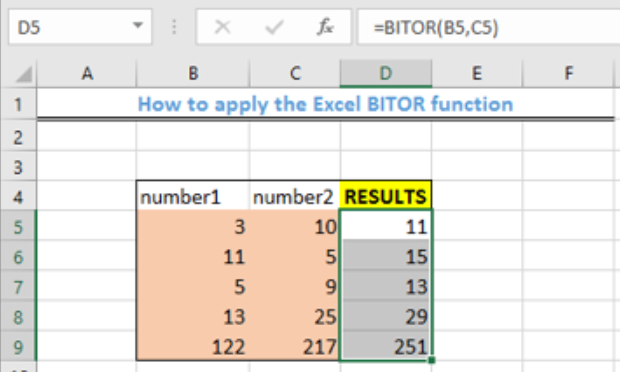Figure 1- Final result of the Excel BITOR function

## General Formula

`=BITOR (num1, num2)`

• Num1: first positive decimal value
• Num2: second positive decimal value

## Formula

`=BITOR(B5,C5)`

## Setting up the data

We will prepare the table as shown in figure 2 with the numerical values. The objective is to find the Bitwise “OR” of the combination of the numbers contained in each row.

• We will input the numerical values for “number1” in the range B5:B9
• We will also enter the numerical figures for “number2” in the range C5:C9
• The result will be displayed in Column D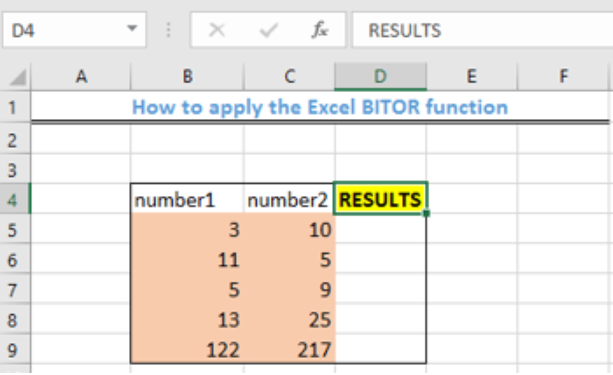Figure 2 – Setting up the data

## Applying the BITOR Function

• We will click on Cell D5
• We will input the formula below into Cell D5

`=BITOR(B5,C5)`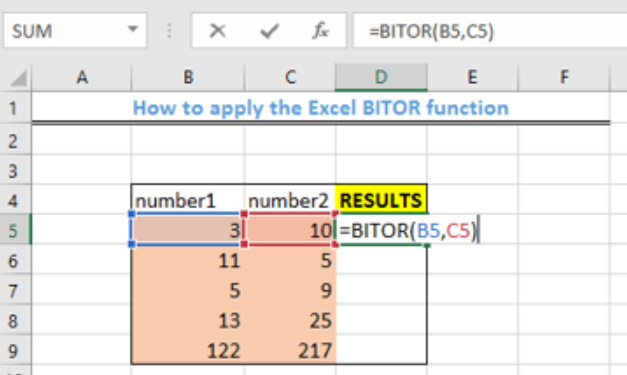Figure 3 – Enter the BITOR logical operation

• We will press the enter key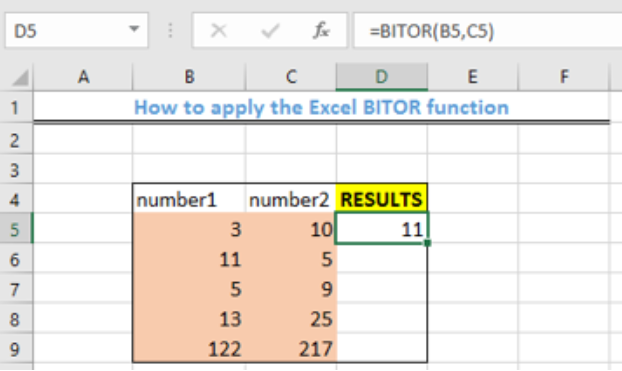Figure 4 – Applying the BITOR function

• We will use the fill handle (the small square box at the bottom right of the cell) to copy the formula to the other cells. Click and drag the fill handle to Cell D9.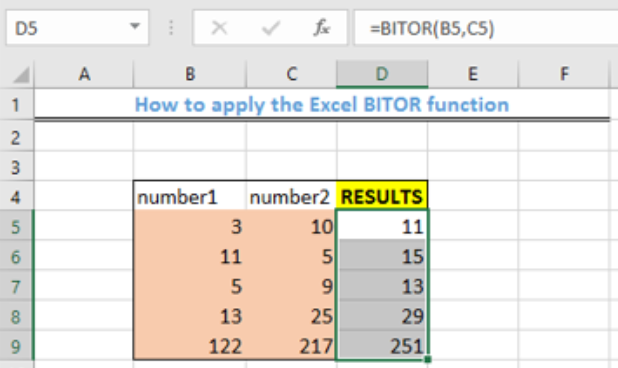Figure 5- Final Result of using the BITOR function

## Explanation

`=BITOR (num1, num2)`

This function adds the binary version of two numbers. This function performs a logical OR operation for the binary representations of each number before addition, and finally, the result is displayed as a number.

For example, in our table, the binary representation for Cell B5 is 11 and Cell C5 is 1010.

Adding their binary representations, we will have:

1010

11

1011

Next, we convert, the binary representation back to a numerical value, by starting from the right using the process below:

1011: 1 X 23 + 0 X 22 + 1 X 21 + 1 X 20 = 11.

The arguments, number 1 and number 2 must always be greater than or equal to zero. Also, it should never be larger than 2^48-1.

## Instant Connection to an Expert through our Excelchat Service

Most of the time, the problem you will need to solve will be more complex than a simple application of a formula or function. If you want to save hours of research and frustration, try our live Excelchat service! Our Excel Experts are available 24/7 to answer any Excel question you may have. We guarantee a connection within 30 seconds and a customized solution within 20 minutes.